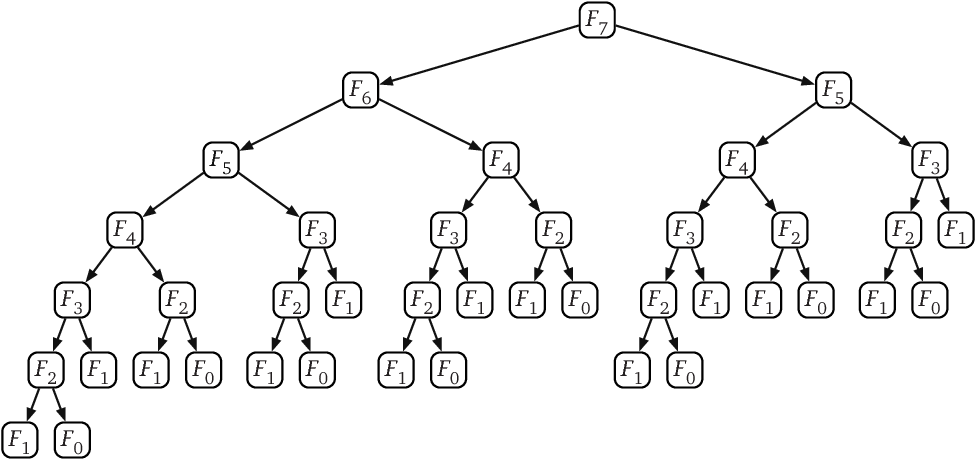# Python中的遞迴

## 費氏數列

1、1、2、3、5、8、13、21……

$$f(1) = f(2) = 1$$
$$f(x) = f(x - 1) + f(x - 2)$$

## Python中的遞迴

$$f(1) = f(2) = 1$$

def f(x):
if x == 1 or x == 2:
return 1

$$f(x)=f(x-1)+f(x-2)$$

def f(x):
if x == 1 or x ==2:
return 1
else:
return f(x-1) + f(x-2)

for i in range(1, 11):
print(f(i))

1
1
2
3
5
8
13
21
34
55

## 遞迴的效能問題

print(f(150))

def f(x):
if x == 1 or x ==2:
return 1
else:
return f(x-1) + f(x-2)def f(x):
x -= 1
curr = [1, 1]
if x == 0 or x == 1:
return curr[x]
for i in range(2, x + 1):
curr.append(curr[i - 1] + curr[i - 2])
return curr[x]

+1